# Automatic continuity for Banach algebras

The basic question in automatic continuity theory is the following. Letandbe Banach algebras (cf. Banach algebra), and let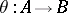be a homomorphism. What algebraic conditions onand/orensure that the homomorphismis automatically continuous? A variation of the question is the following. Letbe a Banach algebra, letbe a Banach-bimodule, and letbe a derivation (cf. also Derivation in a ring). What algebraic conditions onand/orensure thatis automatically continuous? There are important generalizations of the latter question: for various purposes it is important to replace the derivation by the more general notion of an "intertwining mapping" . A special case of the automatic continuity problem for homomorphisms is the uniqueness-of-norm problem, which asks which Banach algebras have a unique complete algebra norm. For a substantial recent (as of 2000) account of automatic continuity theory for Banach algebras, see [a4]; all the terms that are used here are defined in [a4].

The starting point for automatic continuity theory is the easily proved fact that every character on a Banach algebra(i.e., every homomorphism fromonto the complex field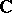) is automatically continuous (cf. also Continuous function). This was already stated in the seminal work of I.M. Gel'fand around 1940. Note that there is a deep related question. Letbe a Fréchet algebra (so that the topology ofis given by a sequence of algebra semi-norms on, andis complete). Then it is an open question (as of 2000) whether or not every character onis automatically continuous. This is called Michael's problem, because it was raised in [a12]. A positive result is known in many cases; a striking sufficient condition, involving analytic functions of several complex variables, for the continuity of all such characters is given in [a7].

It follows easily from the continuity of characters that every homomorphismfrom a Banach algebrainto a commutative semi-simple Banach algebrais continuous. A closely related result is Johnson's uniqueness-of-norm theorem: Every semi-simple Banach algebra has a unique complete algebra norm. For lovely alternative proofs of this theorem, see [a1] and [a13]. There are non-semi-simple commutative Banach algebras having a unique complete algebra norm. For example, this is true of the convolution algebras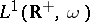, whereis a weight function on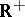(see [a4], § 5.2). On the other hand, there are even commutative Banach algebras with a one-dimensional (Jacobson) radical which do not have a unique complete algebra norm (see [a4], § 5.1). Nevertheless there are striking open questions in this area: it is not known (as of 2000) whether a commutative Banach algebra which is an integral domain necessarily has a unique complete algebra norm; the question is also open for Banach algebras with a finite-dimensional radical; for partial results, see [a5]. The following question is also open. Letandbe Banach algebras, and letbe a homomorphism. Suppose thatis semi-simple and that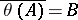. Isautomatically continuous?

The separating space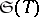of a linear mapping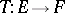, where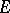and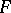are Banach spaces, is defined to be the set of elements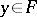such that there is a sequenceinwithinand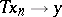in. Clearly,is a closed linear subspace ofand, by the closed-graph theorem,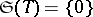if and only ifis continuous. A key result in automatic continuity theory is the stability lemma. One version of this is as follows; there are many variations. Letandbe Banach spaces, letbe a linear mapping, letbe a sequence of Banach spaces with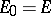, and let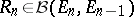(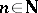). Then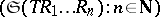is a nest inthat stabilizes. This leads to a proof [a11] that all derivations from a semi-simple Banach algebra to itself are automatically continuous, and to many other results.

Letbe a Banach algebra, letandbe Banach-bimodules, and letbe a linear mapping. The continuity idealofis defined to be. This is an ideal in, and the "bigger IT is, the more continuous T is" . This leads to proofs that all derivations from various Banach algebrasinto each Banach-bimodule are automatically continuous; see [a4], § 5.3. For example, this is true wheneveris a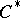-algebra, [a14].

Letbe a homomorphism between Banach algebras. The main boundedness theorem of W.G. Bade and P.C. Curtis Jr. [a2] asserts that, in the case wherehas many idempotents, the continuity idealis necessarily "large" . This leads to a proof that every homomorphism from many algebras, including the algebra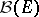of all bounded linear operators on a Banach spacefor certain Banach spaces, is automatically continuous.

Letbe the commutative-algebra of all continuous functions on a compact space, taken with the uniform norm on. The theory of Bade and Curtis shows that each homomorphism frominto a Banach algebra must be continuous on a dense subalgebra of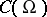; they left open the question of whether such a homomorphism is necessarily continuous on the whole of. Eventually it was proved [a3], [a8] (see [a4], § 5.7) that this is not the case: For each infinite compact space, there is a discontinuous homomorphism frominto certain commutative Banach algebras. Indeed, it is known just which Banach algebras arise in this situation. Note that the proof of this theorem requires the assumption of the continuum hypothesis CH; that some additional set-theoretic hypothesis is required is a remarkable result that is discussed in [a6]. In fact, there is a discontinuous homomorphism from "most" , perhaps all, infinite-dimensional, commutative Banach algebras. It is an attractive result of J.R. Esterle [a9] that all epimorphisms fromonto a Banach algebra are automatically continuous; the analogous question for-algebras is open (as of 2000).

Letbe a locally compact group, and let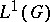be the corresponding group algebra. It is an active area of research to determine whether or not all derivations frominto a Banach-bimodule are automatically continuous. That this is true for many such groupsis proved in [a4], §5.6; a key paper on which this work is based is [a15]. It is a challenging open question (as of 2000) to determine whether or not this is true for all groups, or even for all discrete groups, in which case the corresponding group algebra is denoted by.